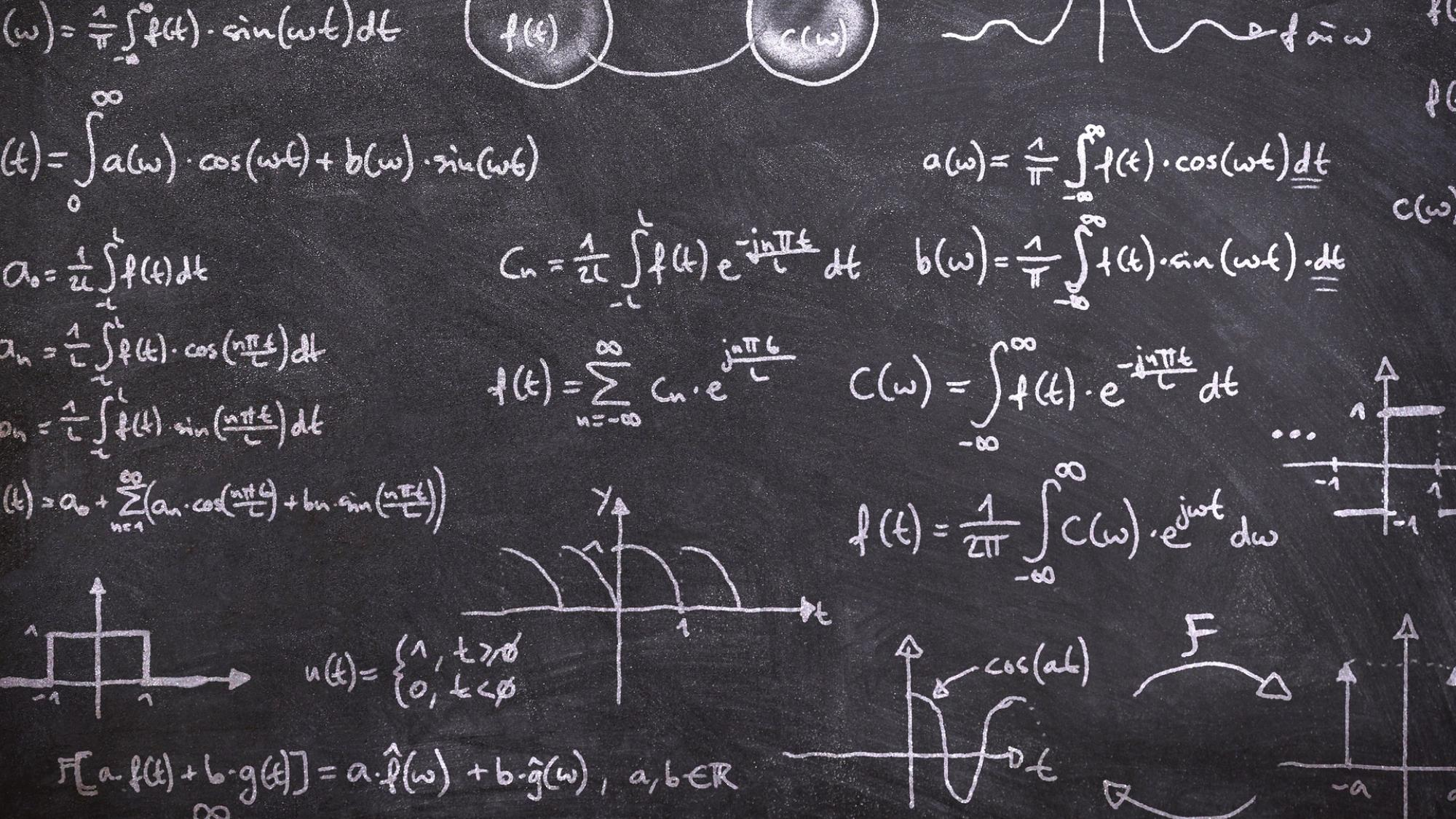# Applied and Computational Mathematics

Applied and Computational Mathematics (ACM) conducts high quality research on different fields of applied and computational mathematics and provides high-class mathematics courses for different faculties, especially for engineering studies.## Research

Applied and Computational Mathematics carries out research in applied mathematics and computational methods, aiming at producing fundamental new knowledge on mathematical tools used in engineering sciences in particular. Emphasis is on both theoretical and numerical analysis of problems modelled by partial differential equations (PDE), on mathematics of computation and algorithms in large scale matrix and operator theory. Also graph theory is investigated.

In PDE, the areas studied are eigenvalue problems associated with waveguides, numerical methods (with FEM, BEM and wavelets) for eigenvalue problems and related spectral estimates. Also theoretical and computational analysis of non-local PDE is conducted, primarily in studies related with fractional diffusion equations. Inverse problems related to PDEs are investigated as well. Of particular interest in this direction are inverse scattering problems where one tries to recover an unknown scatterer from indirect and noisy measurements.

## Teaching

ACM provides both pedagogically and technically high-quality mathematical courses for different faculties. The most of the courses can be taken online wherever you want. Our aim is to create a reasonable and smooth path through the mathematical studies needed.

### Title Spring

You can enroll in the courses by using Tuudo.

## We

ACM is quite a small group but almost all of the engineering students meet someone, or most probably a couple of us during their studies. Don't hesitate to meet or contact us otherwise, if you have something to ask, need more information or want to cooperate with us.